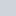# How Can I Remove or Delete Empty Array Elements in PHP?

Author — Nitish Kumar

Many times, when working on a PHP project, you deal with an array which has some unwanted or empty array elements. This is especially true if you have no control over the values populated into the array. For example, you might have to store user input into an array and some of that input might contain unwanted empty strings. In this tutorial, you will learn about different methods which can be used to remove empty elements from an array. We will also learn how to remove other elements with a specific value.

## Remove All Array Elements Which Evaluate to False Using array_filter()

The array_filter() function is used to filter the elements of an array using a callback function. However, this callback function is optional. If you want to remove all array elements whose value evaluates to false, providing a callback function is completely unnecessary.

Without a callback function, `array_filter()` Will remove all elements like the empty string `''`, Boolean false, null and zero etc. Basically, any array element whose value evaluates to false will be removed.

PHP

``````\$input = array('Alex', '', 10, 0, -1, false, null, '0', 'false');

print_r(array_filter(\$input));
/* Output —
Array
(
 => Alex
 => 10
 => -1
 => false
) */``````

In the above example, `array_filter()` removed all array elements which evaluated two false. This includes `''`, `0`, `false`, `null` and `'0'`. One thing that you might have noticed is that the keys of the original array why not re-indexed after removing empty array elements. If you want to re-index the keys, you need to wrap the result of `array_filter()` in the `array_values()` function.

PHP

``````\$input = array('Alex', '', 10, 0, -1, false, null, '0', 'false');

print_r(array_values(array_filter(\$input)));
/* Output —
Array
(
 => Alex
 => 10
 => -1
 => false
) */``````

## Remove Empty Array Elements Using array_filter()

Depending on the needs of current project, the Boolean false value may or may not be considered empty. Similarly, the value 0 may not be considered to be empty as well. If all you want to do is remove empty strings from an array, you will have to pass a custom callback function to `array_filter()`. An element would be removed if the callback function returns false for that particular element. Here is an example:

PHP

``````\$input = array('Alex', '', 10, 0, -1, false, null, '0', 'false');

var_dump(array_filter(\$input, function(\$value) { return \$value !== ''; }));
/* Output —
array(8) {
=>
string(4) "Alex"
=>
int(10)
=>
int(0)
=>
int(-1)
=>
bool(false)
=>
NULL
=>
string(1) "0"
=>
string(5) "false"
} */``````

As you can see in the above example, the only array element that was removed is the empty string. Again, the keys of the final array can be re-indexed using the `array_value()` function.

Another method to remove empty string from an array includes passing the `strlen()` function as a callback to the `array_filter()`. It is important to remember that this only works as expected if the array elements don’t have a Boolean `false` value.

In some cases, an array element will not be an empty string but it will only contain whitespace characters effectively making in empty. If you want to remove all elements which contain either an empty string or a string with only whitespace characters, you will also have to apply `trim()` on all elements in the callback function.

PHP

``````\$input = array('Alex', '', 'Amanda ', '  ', '0', ' Joshua ');

var_dump(array_filter(\$input, function(\$value) { return trim(\$value) !== ''; }));
/* Output —
array(4) {
=>
string(4) "Alex"
=>
string(7) "Amanda "
=>
string(1) "0"
=>
string(8) " Joshua "
} */``````

## Removing Empty Array Elements by Looping Over the Array

You can also loop over an array to remove all elements which are empty or have some other specific value that you want gone. When looking over an array it is important to remember that you have to insert the value of original array instead of a local variable created by the `foreach` loop. The following example will make this concept clearer.

PHP

``````\$input = \$input = array('Alex', '', false, null, '0', 'false');

/* This Method won't Work */
foreach(\$input as \$elem) {
if(\$elem === '') {
unset(\$elem);
}
}

var_dump(\$input);
/* Output —
array(6) {
=>
string(4) "Alex"
=>
string(0) ""
=>
bool(false)
=>
NULL
=>
string(1) "0"
=>
string(5) "false"
} */

/* This Method will Work */
foreach(\$input as \$key => \$value) {
if(\$value === '') {
unset(\$input[\$key]);
}
}

var_dump(\$input);
/* Output —
array(5) {
=>
string(4) "Alex"
=>
bool(false)
=>
NULL
=>
string(1) "0"
=>
string(5) "false"
} */``````

In the first case, the `foreach` loop unsets the local variable called `\$elem`. This is the reason the original arrays stays intact. On the other hand, the second loop uses `unset()` to remove array elements pointed out by the current value of `\$key` in the `\$input` array.

## Quick Summary

Let’s recap everything we have covered in this tutorial.

1. You can simply use `array_filter()` to remove all array elements which evaluate to false. In this case, you don’t need to pass any callback function.
2. Different projects may have different criteria for determining what constitutes an empty array element. In such cases, you can pass a callback function to the `array_filter()` function and remove elements accordingly.
3. If you don’t want to use the `array_filter()` function for some reason, you can also loop over the array to remove any empty array element.

Let me know if there is anything that you would like me to clarify in this tutorial. Also, you are more than welcome to comment if you know other techniques to remove empty array elements in PHP.

Rate this post —(No Ratings Yet)Loading...

Tags: |

Subscribe
Notify of